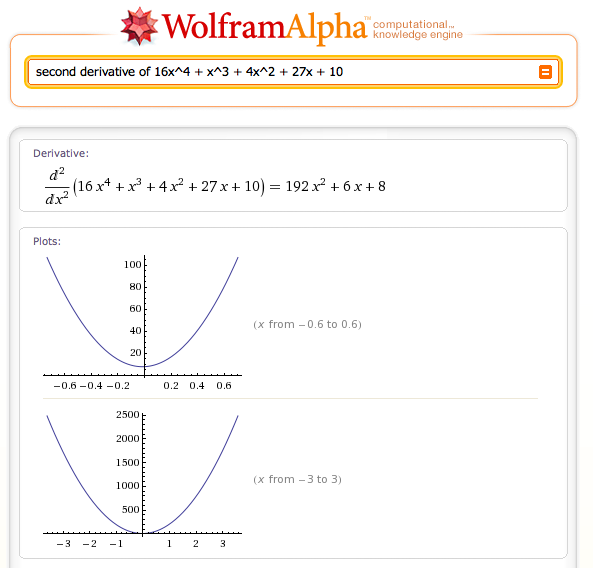## Tuesday, January 19, 2010

### Recollecting The Calculus

Allow me to summarize my recollections of The Calculus:

1. One must always refer to it as "The Calculus," never just "calculus."
2. The first derivative is the instantaneous rate of change, i.e. the velocity.
3. The second derivative is the instantaneous rate of change in the velocity, i.e. accelleration. Etc, etc.
4. Laplace transforms are a much easier way to compute derivatives.
5. I have no recollection of how to use the Laplace transform.

Thats pretty much it, in a nutshell. I suspect that had the modern web existed back then, my calculus classes would have been different but my retention of the material decades later would be about the same.